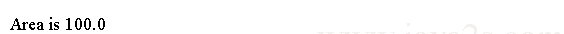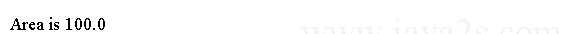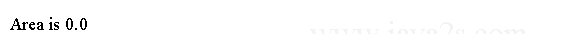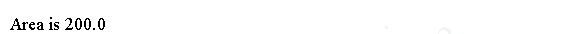# Java Tutorial - Java Constructors

A constructor initializes an object during object creation when using new operator.

Java allows objects to initialize themselves when they are created. This automatic initialization is performed through the use of a constructor.

## Syntax

It has the same name as the class. Constructors have no return type, not even void.

```class ClassName{

ClassName(parameter list){ // constructor
...
}
}
```

In the following code the `Rectangle` class in the following uses a constructor to set the dimensions:

```
class Rectangle {
double width;//from  w w  w  .j a v  a  2s .  c  o  m
double height;

Rectangle() {
width = 10;
height = 10;
}

double area() {
return width * height;
}
}

public class Main {
public static void main(String args[]) {
Rectangle mybox1 = new Rectangle();
double area;
area = mybox1.area();
System.out.println("Area is " + area);

}
}
```

When this program is run, it generates the following results:## Java Default Constructor

A default constructor is a constructor with no parameters.

## Syntax for Java Default Constructor

Syntax for Java Default Constructor

```class ClassName{

ClassName(){ // default constructor
...
}
}
```

In the following code the constructor `Rectangle()` is the default constructor.

```
class Rectangle {
double width;//from  w  ww  .j ava 2 s  .  c om
double height;

Rectangle() {
width = 10;
height = 10;
}

double area() {
return width * height;
}
}

public class Main {
public static void main(String args[]) {
Rectangle mybox1 = new Rectangle();
double area;
area = mybox1.area();
System.out.println("Area is " + area);

}
}
```

If you don't declare a default constructor the Java compiler will add one for you. When you call the default constructor added by Java compiler the class member variables are initialized by default value. If you do provide a default constructor the Java compiler would not insert one for you.

The code above generates the following result.## Example

In the following code we removes the default constructor from class Rectangle. When we compile the class Java compiler adds the default constructor for us so we can still construct a Rectangle object by calling the default constructor. But the value of `width` and `height` would be initialized to `0.0`.

```
class Rectangle {
double width;/*from  w w w.j  a  v  a2  s  .  c o  m*/
double height;

double area() {
return width * height;
}
}

public class Main {
public static void main(String args[]) {
Rectangle mybox1 = new Rectangle();
double area;
area = mybox1.area();
System.out.println("Area is " + area);

}
}
```

The output:## Java Constructor Parameters

The constructors can also have parameters. Usually the parameters are used to set the initial states of the object.

## Syntax for Java Constructor Parameters

Syntax for Java Constructor Parameters

```class ClassName{

ClassName(parameterType variable,parameterType2 variable2,...){ // constructor
...
}
}
```

In the the following demo code `Rectangle` class uses the parameters, `w` for width and `h` for height, from the constructors to initialize its width and height.

```
class Rectangle {
double width;// w  ww .j  a v a  2  s. com
double height;

Rectangle(double w, double h) {
width = w;
height = h;
}

double area() {
return width * height;
}
}

public class Main {
public static void main(String args[]) {
Rectangle mybox1 = new Rectangle(10, 20);
double area;
area = mybox1.area();
System.out.println("Area is " + area);
}
}
```

The output from this program is shown here:## Example 2

Just like methods in a class the constructors can not only accept primitive type parameters it can also have the object parameters. Object parameters contains more information and can help us initialize the class.

The following `Rectangle` class has a constructor whose parameter is a `Rectangle` class. In this way we can initialize a rectangle by the data from another rectangle.

```
class Rectangle {
double width;/* w w w.  jav a 2s . c o m*/
double height;

Rectangle(Rectangle ob) { // pass object to constructor
width = ob.width;
height = ob.height;
}

Rectangle(double w, double h) {
width = w;
height = h;
}

// constructor used when no dimensions specified
Rectangle() {
width = -1; // use -1 to indicate
height = -1; // an uninitialized
}

// constructor used when cube is created
Rectangle(double len) {
width = height = len;
}

double area() {
return width * height;
}
}

public class Main {
public static void main(String args[]) {
Rectangle mybox1 = new Rectangle(10, 20);
Rectangle myclone = new Rectangle(mybox1);
double area;
// get volume of first box
area = mybox1.area();
System.out.println("Area of mybox1 is " + area);
// get volume of clone
area = myclone.area();
System.out.println("Area of clone is " + area);

}
}
```

The output:Method overloading is to declare two or more methods with the name but different type or count of parameters.

In the following code `Rectangle` defines three constructors to initialize the dimensions of a rectangle in various ways.

```
class Rectangle {
double width;//  ww w . j  a  va  2  s .  c  o  m
double height;

// constructor used when all dimensions specified
Rectangle(double w, double h) {
width = w;
height = h;
}

// constructor used when no dimensions specified
Rectangle() {
width = -1; // use -1 to indicate
height = -1; // an uninitialized
}

Rectangle(double len) {
width = height = len;
}

double area() {
return width * height;
}
}

public class Main {
public static void main(String args[]) {
Rectangle mybox1 = new Rectangle(10, 20);
Rectangle mybox2 = new Rectangle();
Rectangle mycube = new Rectangle(7);

double area = mybox1.area();
System.out.println(area);

area = mybox2.area();
System.out.println(area);

area = mycube.area();
System.out.println(area);

}
}
```

The output produced by this program is shown here:## this()

In Java `this` keyword can call the overloaded constructors. The general form is shown here:

`this(arg-list)`

When `this()` is executed, the overloaded constructor that matches the arg-list is executed first.

The call to `this()` must be the first statement within a constructor.

The following code defines a class named MyClass. It has three constructors. The first constructor accepts two int values. The second accepts one int type value. The third one accepts no value.

```class MyClass {/*from  ww w. j a v a 2 s.  com*/
int a;
int b;

// initialize a and b individually
MyClass(int i, int j) {
a = i;
b = j;
}

// initialize a and b to the same value
MyClass(int i) {
this(i, i); // invokes MyClass(i, i)
}

// give a and b default values of 0
MyClass() {
this(0); // invokes MyClass(0)
}
}
```